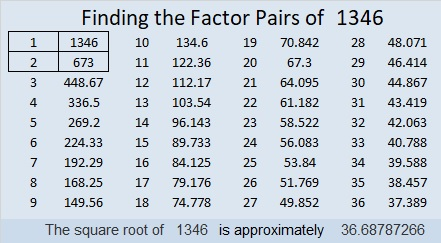# (1346÷2)×3 = 2019 Magic or Square?

I was inspired to make a 3 × 3 Magic Square where every number is different but the numbers in each row, column and diagonal added together equal the same number, 54:I made it by taking a regular 3 × 3 Magic Square and adding 13 to each of its numbers.

What inspired me to do that? This magical tweet of a palindromic Magic Square for the Year 2019:

Yeah, I know my magic square isn’t quite as impressive. It might be more square than it is magic. It’s also less impressive than this number 2019 spelled out using fifty-one
4 × 4 Magic Squares.

Nevertheless, I made it, and I’m sharing it in this post I’ve numbered 1346, a special number this year because (1346÷2)×3 = 2019

Here’s more about the number 1346:

• 1346 is a composite number.
• Prime factorization: 1346 = 2 × 673
• 1346 has no exponents greater than 1 in its prime factorization, so √1346 cannot be simplified.
• The exponents in the prime factorization are 1, and 1. Adding one to each exponent and multiplying we get (1 + 1)(1 + 1) = 2 × 2 = 4. Therefore 1346 has exactly 4 factors.
• The factors of 1346 are outlined with their factor pair partners in the graphic below.1346 is the hypotenuse of a Pythagorean triple:
770-1104-1346 which is 2 times 385-552-673